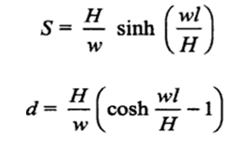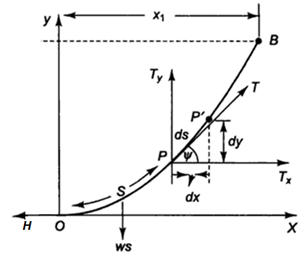# Sag of Transmission Line with supports at same height Calculator

This Calctown Calculator calculates the sag of a transmission line with supports at the same height.

N
kg/m
m

#### Result

m
mwhere

S = length of line from point O

d = vertica distance of end points from O

w = weight of line per unit length

H = tension at point O

2l = span length

O is the lowest point on the line